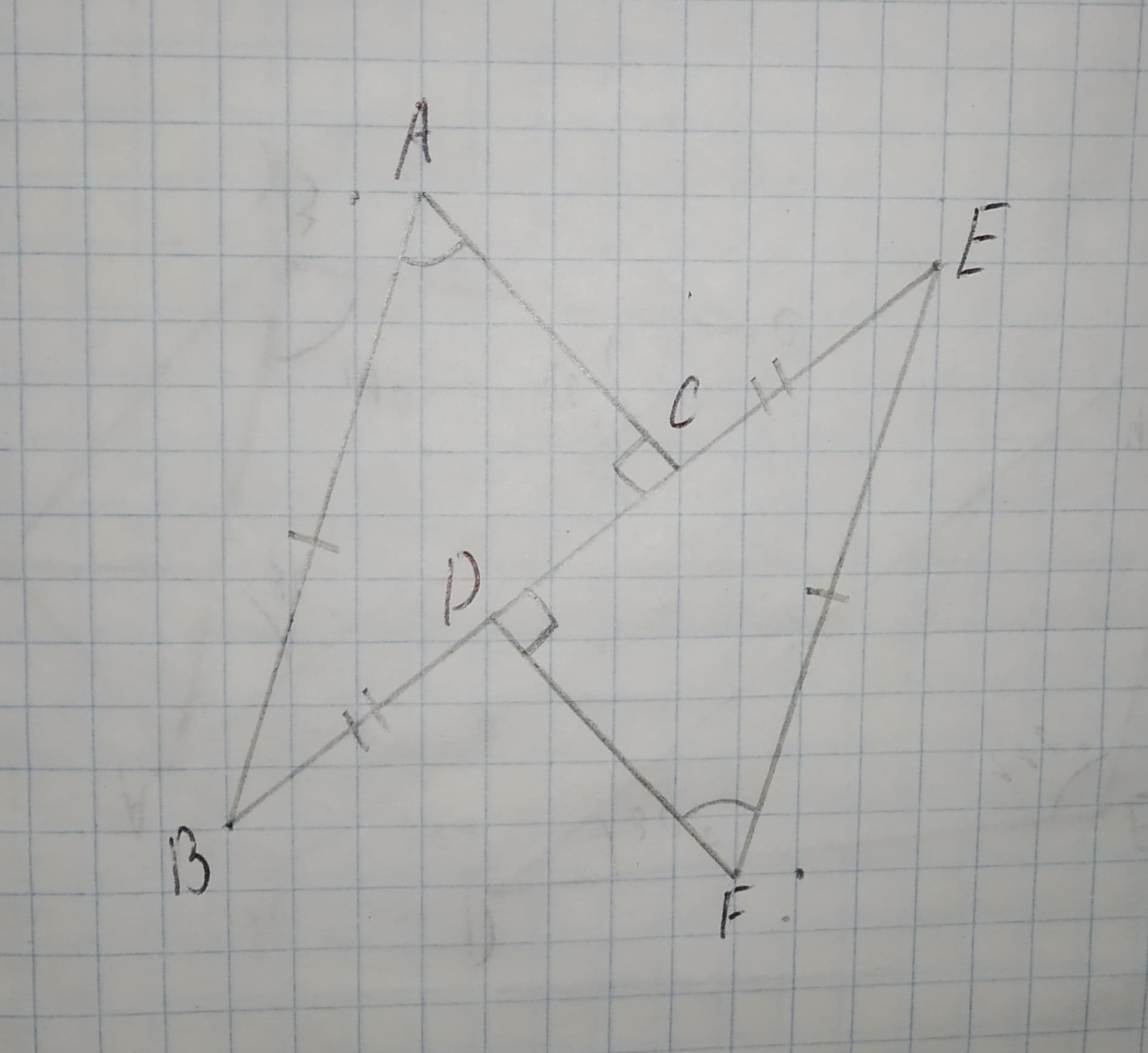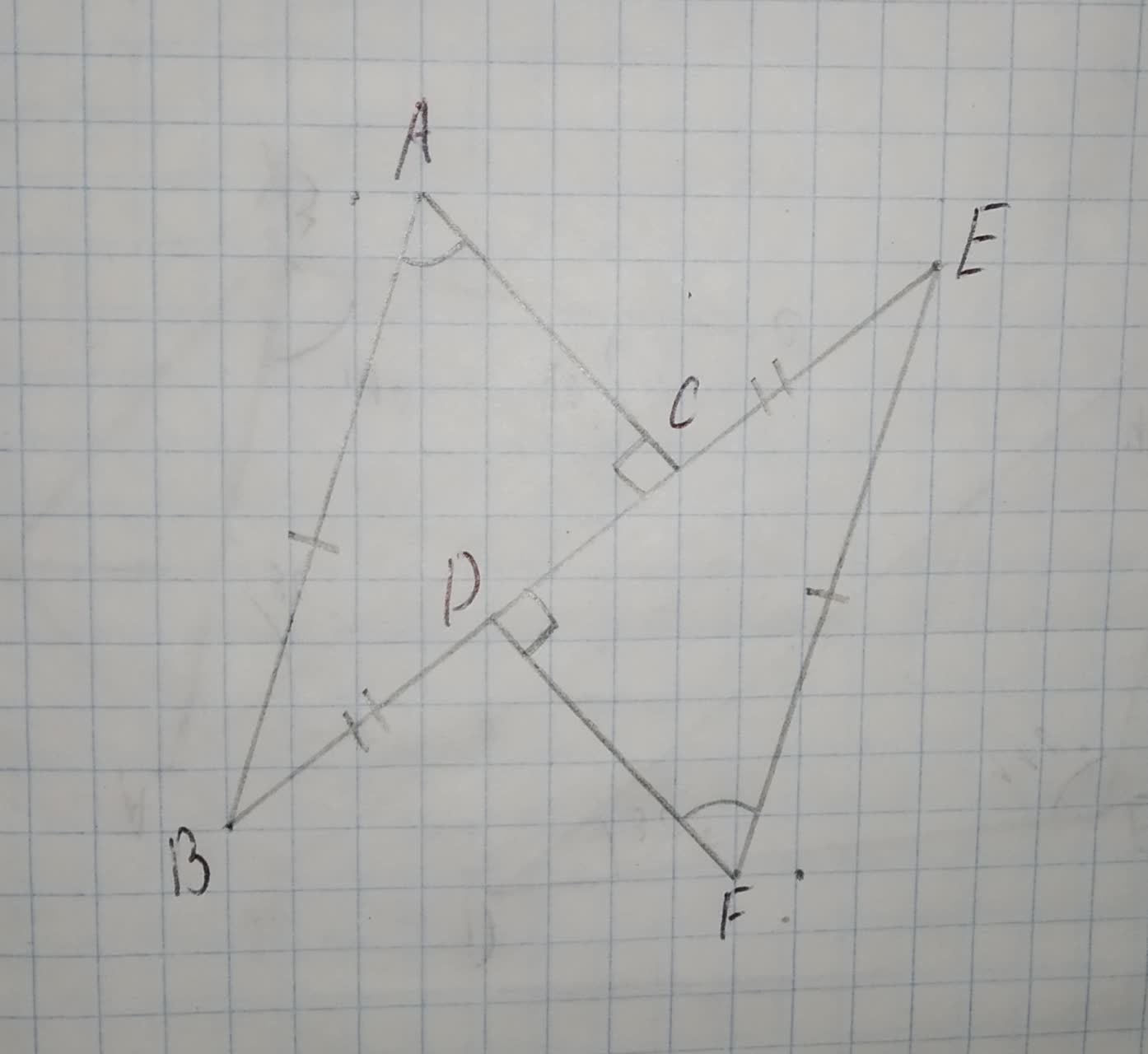# Decide whether enough information is given to prove that the triangles are congruent using the SAS Congruence Theorem.12210203322.jpglwfrgin 2020-12-16 Answered

Decide whether enough information is given to prove that the triangles are congruent using the SAS Congruence Theorem.You can still ask an expert for help

## Want to know more about Congruence?

• Questions are typically answered in as fast as 30 minutes

Solve your problem for the price of one coffee

• Math expert for every subject
• Pay only if we can solve itBrittany Patton

Step 1
To make the triangle congruent by using the SAS congruence,
First naming the edges and vertex of the triangle,Step 2
By using sum of interior angle property in $\mathrm{△}ABC\phantom{\rule{1em}{0ex}}\text{and}\phantom{\rule{1em}{0ex}}\mathrm{△}DEF$,
$\mathrm{\angle }ABC={180}^{\circ }-\left(\mathrm{\angle }ABC+\mathrm{\angle }BAC\right)$
$\mathrm{\angle }DEF={180}^{\circ }-\left(\mathrm{\angle }EDF+\mathrm{\angle }DFE\right)$
it is given that,
$\mathrm{\angle }ACB=\mathrm{\angle }EDF={90}^{\circ }$ (given)...(i)
$\mathrm{\angle }BAC=\mathrm{\angle }DFE\left(given\right)$...(ii)
Adding equation (i) and (ii) and subtracting them form ${180}^{\circ }$ in both sides,
${180}^{\circ }-\left(\mathrm{\angle }ACB+\mathrm{\angle }BAC\right)={180}^{\circ }-\left(\mathrm{\angle }EDF+\mathrm{\angle }DFE\right)$
$\mathrm{\angle }ABC=\mathrm{\angle }DEF$
Now, in $\mathrm{△}ABC\phantom{\rule{1em}{0ex}}\text{and}\phantom{\rule{1em}{0ex}}\mathrm{△}DEF$,
AB=EF(given)
$\mathrm{\angle }ABC=\mathrm{\angle }DEF$(Proved above)
BC=DE(given)
$\mathrm{△}ABC\stackrel{\sim }{=}\mathrm{△}DEF$ (By SAS Congruence Theorem)
Yes, the provided information is enough to prove the triangle congruent by SAS.